# RD Sharma Solutions for Class 8 Maths Chapter 23 Data Handling - I (Classification and Tabulation of Data) Exercise 23.1

In Exercise 23.1 of Chapter 23, we shall discuss concepts related to RAW data, presentation of data, frequency distribution (which includes (i) discrete frequency distribution (ii) continuous or grouped frequency distribution). RD Sharma Class 8 Solutions are prepared by our expert tutors, which have all the solutions to problems of this exercise to help students build their problem-solving abilities. To access the solutions offline, students can download the pdf from the links provided below.

## Download the Pdf of RD Sharma Solutions for Class 8 Maths Exercise 23.1 Chapter 23 Data Handling – I (Classification and Tabulation of Data)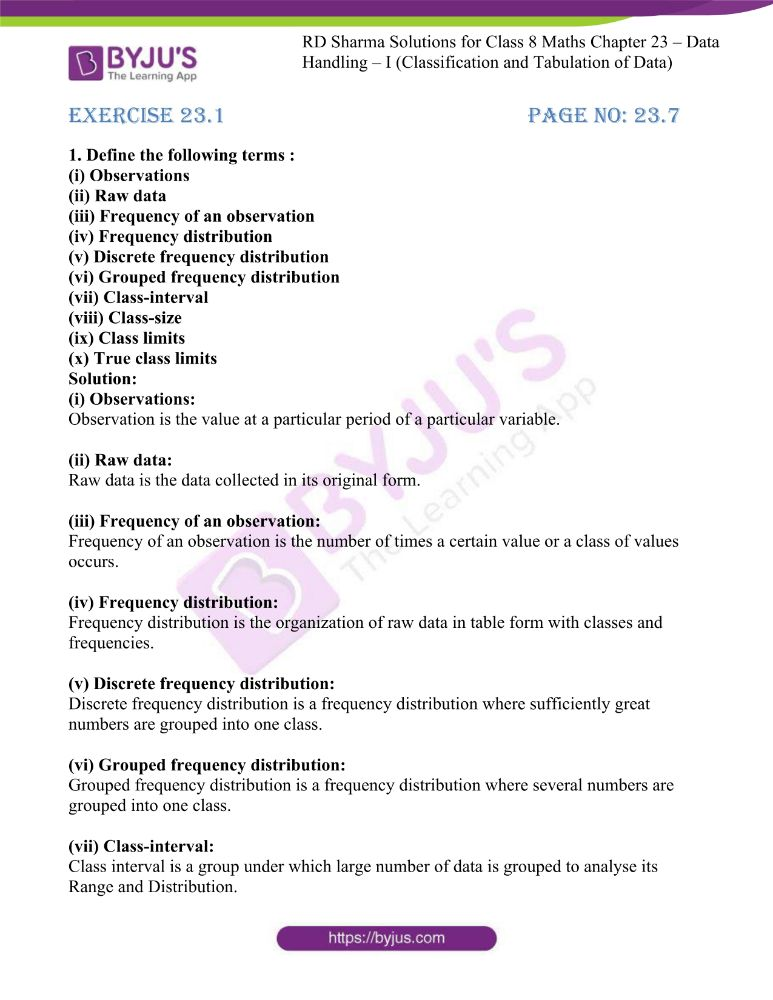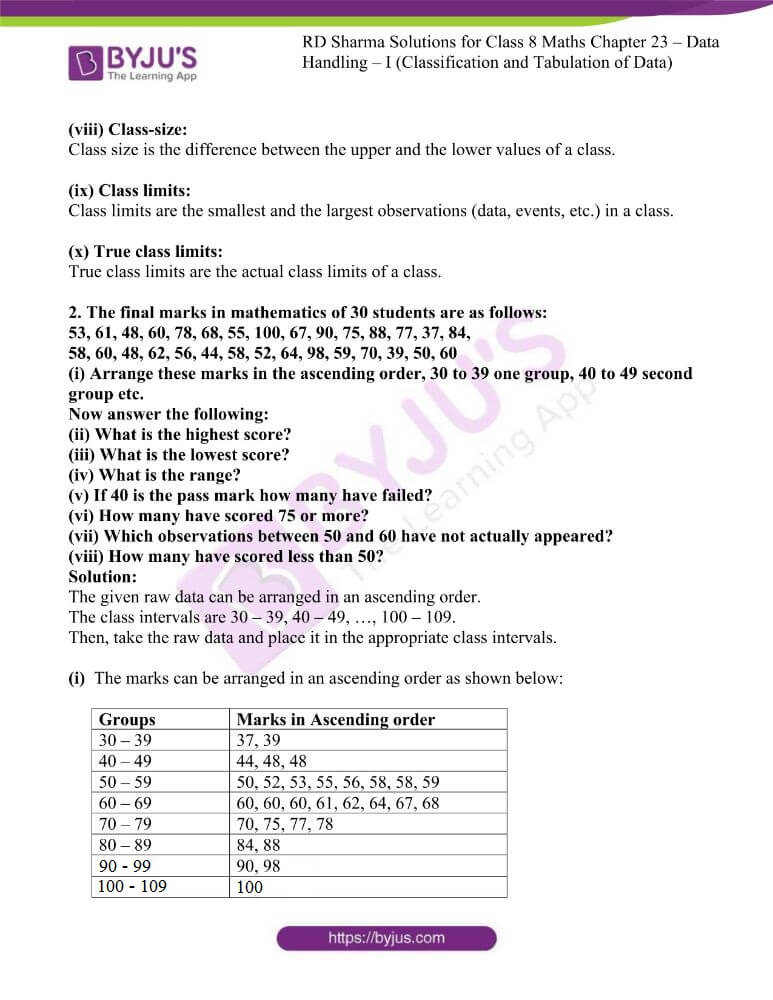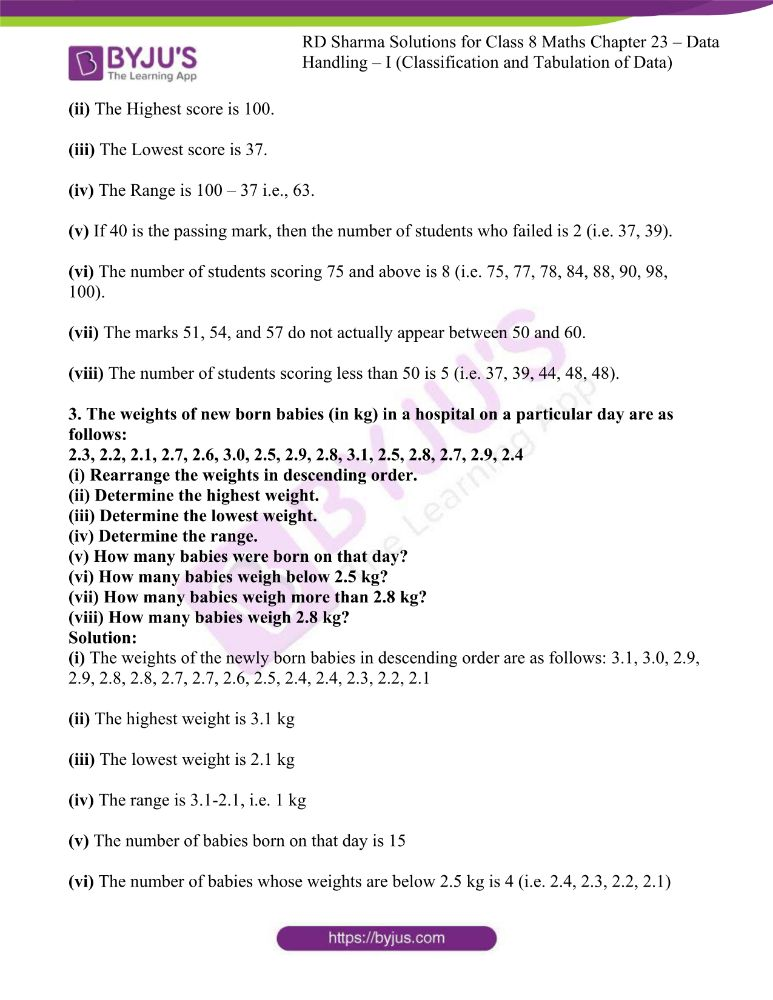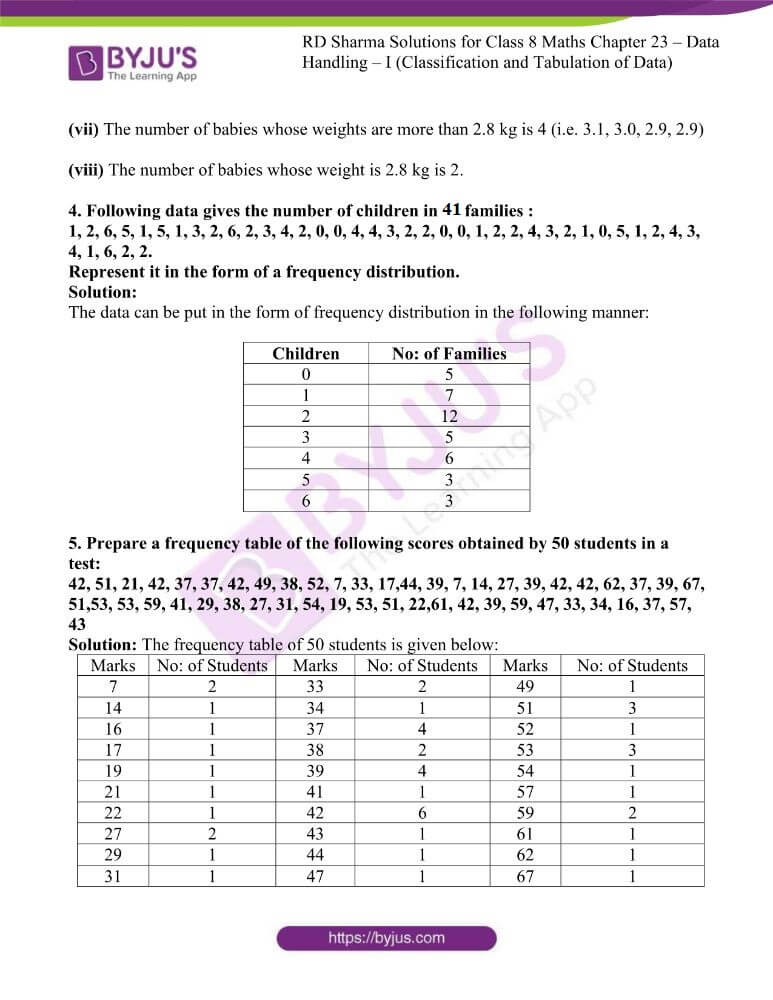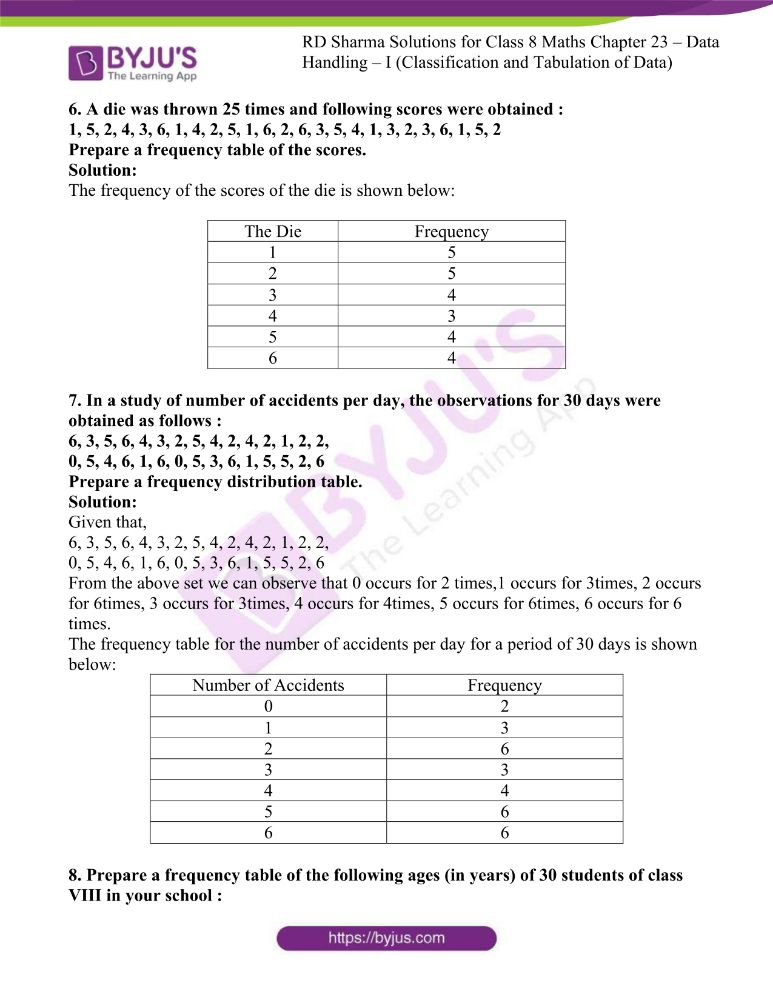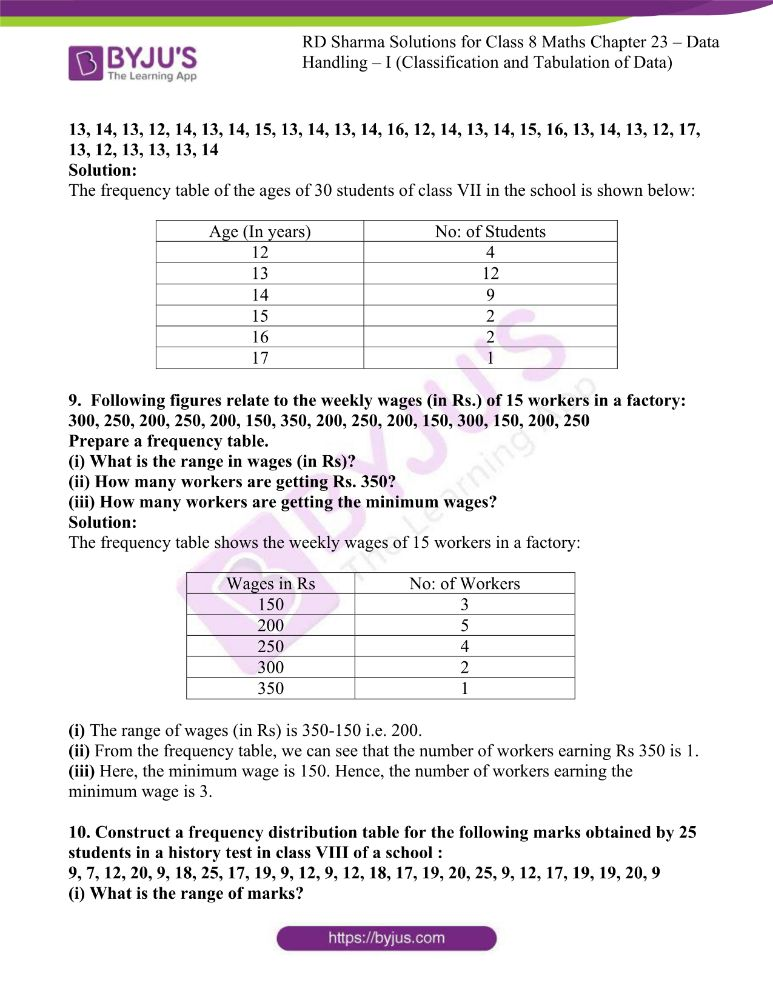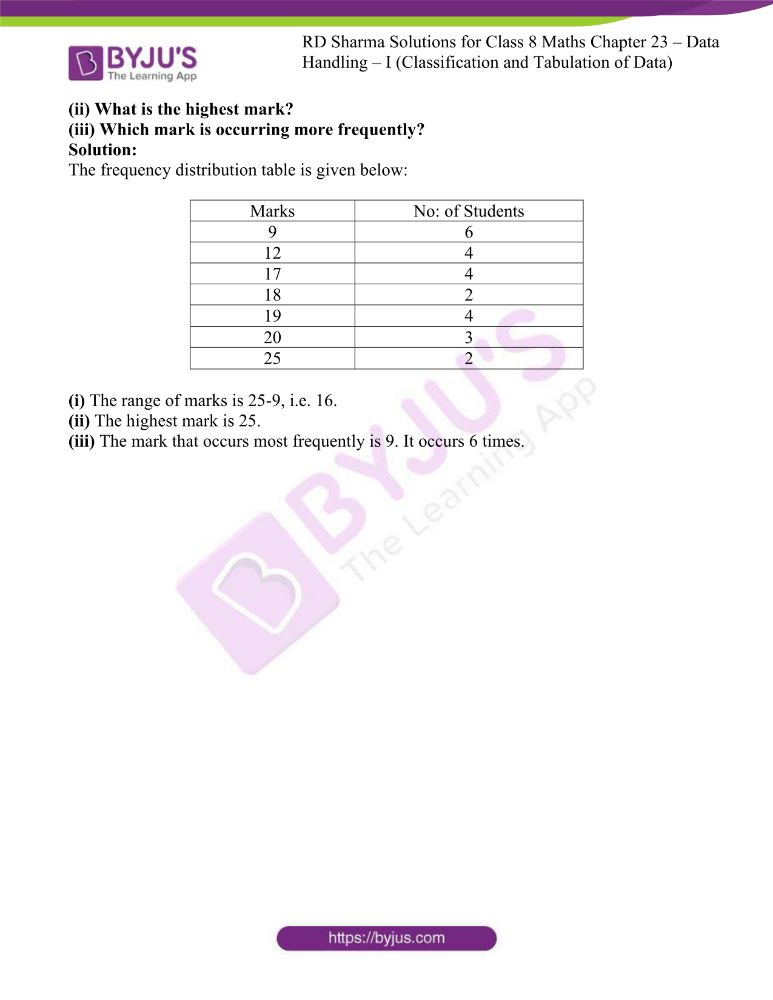### Access answers to RD Sharma Maths Solutions For Class 8 Exercise 23.1 Chapter 23 Data Handling – I (Classification and Tabulation of Data)

1. Define the following terms :
(i) Observations
(ii) Raw data
(iii) Frequency of an observation
(iv) Frequency distribution
(v) Discrete frequency distribution
(vi) Grouped frequency distribution
(vii) Class-interval
(viii) Class-size
(ix) Class limits
(x) True class limits

Solution:

(i) Observations:

Observation is the value at a particular period of a particular variable.

(ii) Raw data:

Raw data is the data collected in its original form.

(iii) Frequency of an observation:

Frequency of an observation is the number of times a certain value or a class of values occurs.

(iv) Frequency distribution:

Frequency distribution is the organization of raw data in table form with classes and frequencies.

(v) Discrete frequency distribution:

Discrete frequency distribution is a frequency distribution where sufficiently great numbers are grouped into one class.

(vi) Grouped frequency distribution:

Grouped frequency distribution is a frequency distribution where several numbers are grouped into one class.

(vii) Class-interval:

Class interval is a group under which large number of data is grouped to analyse its Range and Distribution.

(viii) Class-size:

Class size is the difference between the upper and the lower values of a class.

(ix) Class limits:

Class limits are the smallest and the largest observations (data, events, etc.) in a class.

(x) True class limits:

True class limits are the actual class limits of a class.

2. The final marks in mathematics of 30 students are as follows:
53, 61, 48, 60, 78, 68, 55, 100, 67, 90, 75, 88, 77, 37, 84,
58, 60, 48, 62, 56, 44, 58, 52, 64, 98, 59, 70, 39, 50, 60
(i) Arrange these marks in the ascending order, 30 to 39 one group, 40 to 49 second group etc.
(ii) What is the highest score?
(iii) What is the lowest score?
(iv) What is the range?
(v) If 40 is the pass mark how many have failed?
(vi) How many have scored 75 or more?
(vii) Which observations between 50 and 60 have not actually appeared?
(viii) How many have scored less than 50?

Solution:

The given raw data can be arranged in an ascending order.

The class intervals are 30 – 39, 40 – 49, …, 100 – 109.

Then, take the raw data and place it in the appropriate class intervals.

(i)  The marks can be arranged in an ascending order as shown below:

 Groups Marks in Ascending order 30 – 39 37, 39 40 – 49 44, 48, 48 50 – 59 50, 52, 53, 55, 56, 58, 58, 59 60 – 69 60, 60, 60, 61, 62, 64, 67, 68 70 – 79 70, 75, 77, 78 80 – 89 84, 88 90 – 99 90, 98 100 – 109 100

(ii) The Highest score is 100.

(iii) The Lowest score is 37.

(iv) The Range is 100 – 37 i.e., 63.

(v) If 40 is the passing mark, then the number of students who failed is 2 (i.e. 37, 39).

(vi) The number of students scoring 75 and above is 8 (i.e. 75, 77, 78, 84, 88, 90, 98, 100).

(vii) The marks 51, 54, and 57 do not actually appear between 50 and 60.

(viii) The number of students scoring less than 50 is 5 (i.e. 37, 39, 44, 48, 48).

3. The weights of new born babies (in kg) in a hospital on a particular day are as follows:
2.3, 2.2, 2.1, 2.7, 2.6, 3.0, 2.5, 2.9, 2.8, 3.1, 2.5, 2.8, 2.7, 2.9, 2.4
(i) Rearrange the weights in descending order.
(ii) Determine the highest weight.
(iii) Determine the lowest weight.
(iv) Determine the range.
(v) How many babies were born on that day?
(vi) How many babies weigh below 2.5 kg?
(vii) How many babies weigh more than 2.8 kg?
(viii) How many babies weigh 2.8 kg?

Solution:

(i) The weights of the newly born babies in descending order are as follows: 3.1, 3.0, 2.9, 2.9, 2.8, 2.8, 2.7, 2.7, 2.6, 2.5, 2.4, 2.4, 2.3, 2.2, 2.1

(ii) The highest weight is 3.1 kg

(iii) The lowest weight is 2.1 kg

(iv) The range is 3.1-2.1, i.e. 1 kg

(v) The number of babies born on that day is 15

(vi) The number of babies whose weights are below 2.5 kg is 4 (i.e. 2.4, 2.3, 2.2, 2.1)

(vii) The number of babies whose weights are more than 2.8 kg is 4 (i.e. 3.1, 3.0, 2.9, 2.9)

(viii) The number of babies whose weight is 2.8 kg is 2.

4. Following data gives the number of children in 41 families :
1, 2, 6, 5, 1, 5, 1, 3, 2, 6, 2, 3, 4, 2, 0, 0, 4, 4, 3, 2, 2, 0, 0, 1, 2, 2, 4, 3, 2, 1, 0, 5, 1, 2, 4, 3, 4, 1, 6, 2, 2.
Represent it in the form of a frequency distribution.

Solution:

The data can be put in the form of frequency distribution in the following manner:

 Children No: of Families 0 5 1 7 2 12 3 5 4 6 5 3 6 3

5. Prepare a frequency table of the following scores obtained by 50 students in a test:
42, 51, 21, 42, 37, 37, 42, 49, 38, 52, 7, 33, 17,44, 39, 7, 14, 27, 39, 42, 42, 62, 37, 39, 67, 51,53, 53, 59, 41, 29, 38, 27, 31, 54, 19, 53, 51, 22,61, 42, 39, 59, 47, 33, 34, 16, 37, 57, 43

Solution: The frequency table of 50 students is given below:

 Marks No: of Students Marks No: of Students Marks No: of Students 7 2 33 2 49 1 14 1 34 1 51 3 16 1 37 4 52 1 17 1 38 2 53 3 19 1 39 4 54 1 21 1 41 1 57 1 22 1 42 6 59 2 27 2 43 1 61 1 29 1 44 1 62 1 31 1 47 1 67 1

6. A die was thrown 25 times and following scores were obtained :
1, 5, 2, 4, 3, 6, 1, 4, 2, 5, 1, 6, 2, 6, 3, 5, 4, 1, 3, 2, 3, 6, 1, 5, 2
Prepare a frequency table of the scores.

Solution:

The frequency of the scores of the die is shown below:

 The Die Frequency 1 5 2 5 3 4 4 3 5 4 6 4

7. In a study of number of accidents per day, the observations for 30 days were obtained as follows :
6, 3, 5, 6, 4, 3, 2, 5, 4, 2, 4, 2, 1, 2, 2,
0, 5, 4, 6, 1, 6, 0, 5, 3, 6, 1, 5, 5, 2, 6
Prepare a frequency distribution table.

Solution:

Given that,

6, 3, 5, 6, 4, 3, 2, 5, 4, 2, 4, 2, 1, 2, 2,

0, 5, 4, 6, 1, 6, 0, 5, 3, 6, 1, 5, 5, 2, 6

From the above set we can observe that 0 occurs for 2 times,1 occurs for 3times, 2 occurs for 6times, 3 occurs for 3times, 4 occurs for 4times, 5 occurs for 6times, 6 occurs for 6 times.

The frequency table for the number of accidents per day for a period of 30 days is shown below:

 Number of Accidents Frequency 0 2 1 3 2 6 3 3 4 4 5 6 6 6

8. Prepare a frequency table of the following ages (in years) of 30 students of class VIII in your school :

13, 14, 13, 12, 14, 13, 14, 15, 13, 14, 13, 14, 16, 12, 14, 13, 14, 15, 16, 13, 14, 13, 12, 17, 13, 12, 13, 13, 13, 14

Solution:

The frequency table of the ages of 30 students of class VII in the school is shown below:

 Age (In years) No: of Students 12 4 13 12 14 9 15 2 16 2 17 1

9. Following figures relate to the weekly wages (in Rs.) of 15 workers in a factory:
300, 250, 200, 250, 200, 150, 350, 200, 250, 200, 150, 300, 150, 200, 250
Prepare a frequency table.
(i) What is the range in wages (in Rs)?
(ii) How many workers are getting Rs. 350?
(iii) How many workers are getting the minimum wages?

Solution:

The frequency table shows the weekly wages of 15 workers in a factory:

 Wages in Rs No: of Workers 150 3 200 5 250 4 300 2 350 1

(i) The range of wages (in Rs) is 350-150 i.e. 200.

(ii) From the frequency table, we can see that the number of workers earning Rs 350 is 1.

(iii) Here, the minimum wage is 150. Hence, the number of workers earning the minimum wage is 3.

10. Construct a frequency distribution table for the following marks obtained by 25 students in a history test in class VIII of a school :
9, 7, 12, 20, 9, 18, 25, 17, 19, 9, 12, 9, 12, 18, 17, 19, 20, 25, 9, 12, 17, 19, 19, 20, 9
(i) What is the range of marks?
(ii) What is the highest mark?
(iii) Which mark is occurring more frequently?

Solution:

The frequency distribution table is given below:

 Marks No: of Students 9 6 12 4 17 4 18 2 19 4 20 3 25 2

(i) The range of marks is 25-9, i.e. 16.

(ii) The highest mark is 25.

(iii) The mark that occurs most frequently is 9. It occurs 6 times.